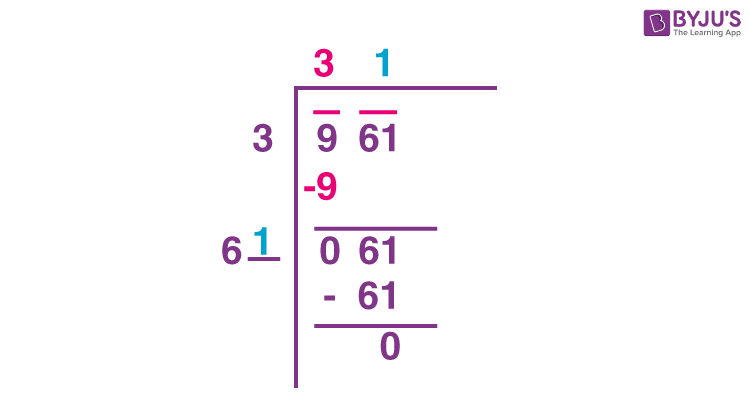# Find square root of 961 by division method.

Solution:

Given number is 961.

Place a bar over every pair of digits starting from the digit at one’s place. If the number of digits in it is odd, then the left-most single digit too will have a bar.

We group the numbers. 9, 61

Then consider 9.

Find the largest number whose square is less than or equal to the number under the

extreme left bar(9). We get 3 here. Take this number as the divisor and the quotient with the number under the extreme left bar as the dividend. Divide and get the remainder

Now the quotient is 3. Remainder is 0.

We bring down 61.

Double the divisor and enter it with a blank on its right. It becomes 6 _.

Guess a largest possible digit to fill the blank which will also become the new digit in the quotient, such that when the new divisor is multiplied to the new quotient the product is less than or equal to the dividend. In this case it is 61×1. So we choose new digit as 1. Get the remainder. Since the remainder is 0 and no digits are left in the given number the square root of 961 is 31.(1)(0)